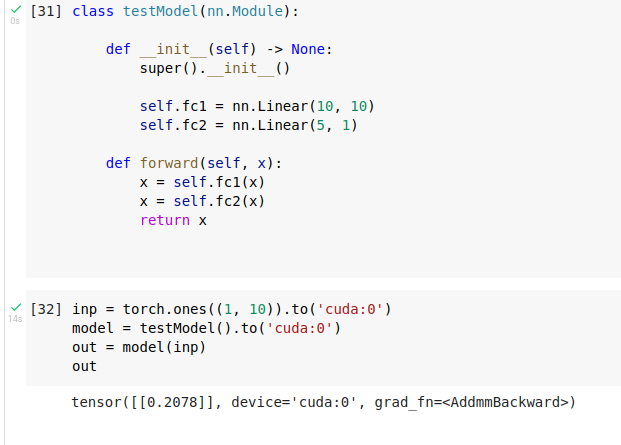# Incorrect Model runs while on GPU!

Hi,

Here is a simple test model, which is incorrect due to size mismatch between fc1 and fc2. Trying to use the model gives a mat size mismatch error as expected. However, on computing the same on a GPU gives an output without any errors!

The model:

``````class testModel(nn.Module):

def __init__(self) -> None:

super().__init__() #Python3 syntax

self.fc1 = nn.Linear(10, 10)
self.fc2 = nn.Linear(5,1)

def forward(self, x):

x = self.fc1(x)
x = self.fc2(x)

return x
``````

This does not work (As expected):

``````inp   = torch.ones((1,10))
model = testModel()
out = model(inp)
``````

The resulting error:
“RuntimeError: mat1 and mat2 shapes cannot be multiplied (1x10 and 5x1)”

But this works!

``````inp   = torch.ones((1,10)).to('cuda:0')
model = testModel().to('cuda:0')
out = model(inp)
``````

I am assuming it has something to do with “has_torch_function_variadic” in nn.functional.

Is this a bug or any reason this is allowed to happen?

Thanks,
Chirag

Hi,
Can you please specify which version of PyTorch you are using?
I can’t reproduce the issue with PyTorch 1.9. I am having the matrix mismatch error, as expected.

``````class testModel(nn.Module):

def __init__(self) -> None:
super().__init__()

self.fc1 = nn.Linear(10, 10)
self.fc2 = nn.Linear(5, 1)

def forward(self, x):
x = self.fc1(x)
x = self.fc2(x)
return x

inp = torch.ones((1, 10)).to('cuda:0')
model = testModel().to('cuda:0')
out = model(inp)
``````

And, the error, as it should be.

``````Traceback (most recent call last):
File "/home/la-belva/.config/JetBrains/PyCharmCE2021.2/scratches/scratch_15.py", line 20, in <module>
out = model(inp)
File "/home/la-belva/anaconda3/envs/latest/lib/python3.8/site-packages/torch/nn/modules/module.py", line 889, in _call_impl
result = self.forward(*input, **kwargs)
File "/home/la-belva/.config/JetBrains/PyCharmCE2021.2/scratches/scratch_15.py", line 15, in forward
x = self.fc2(x)
File "/home/la-belva/anaconda3/envs/latest/lib/python3.8/site-packages/torch/nn/modules/module.py", line 889, in _call_impl
result = self.forward(*input, **kwargs)
File "/home/la-belva/anaconda3/envs/latest/lib/python3.8/site-packages/torch/nn/modules/linear.py", line 94, in forward
return F.linear(input, self.weight, self.bias)
File "/home/la-belva/anaconda3/envs/latest/lib/python3.8/site-packages/torch/nn/functional.py", line 1753, in linear
RuntimeError: mat1 dim 1 must match mat2 dim 0

Process finished with exit code 1

``````Hi,

I’m using torch version 1.9.0+cu102.

Thanks,
Chirag

You are most likely running into a known and already fixed issue described here, so please update your PyTorch version.

1 Like

@Chirag
Can confirm this issue in Colab for Pytorch 1.9.0.And, this issue is resolved after upgrading to PyTorch 1.9.1 as @ptrblck suggested.

Got it! Thanks @Sayed_Nadim , @ptrblck .